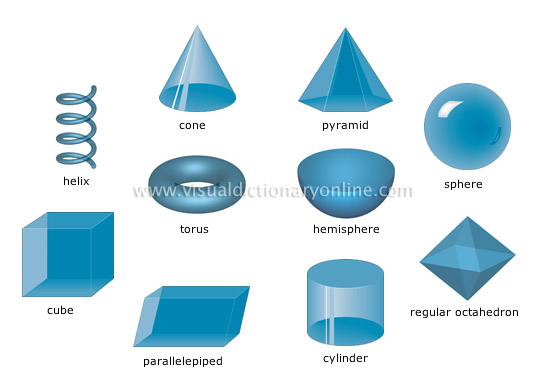Visual Dictionary Online

Theme

solidsGeometric shapes in three dimensions that are delimited by surfaces.helixVolume or solid of spiral shape that turns at a constant angle.

cylinderVolume or solid generated by the rotation of a straight line (generatrix) moving along a curved line (directrix).

regular octahedronVolume or solid with eight triangular sides of equal area; it has six vertices and 12 edges.

parallelepipedVolume or solid with six sides (parallelograms) that are parallel in pairs.

cubeVolume or solid with six square sides of equal area and six equal edges; it has eight vertices.

pyramidVolume or solid generated by straight lines (edges) connecting the angles of a polygon (base) to the vertex and whose sides form triangles.

coneVolume or solid generated by the rotation of a straight line (generatrix) along a circular line (directrix) from a fixed point (vertex).

torusVolume or solid generated by the rotation of a circle at an equal distance from its center of rotation.

sphereVolume with all the points on its surface the same distance from its center; the solid thus delimited is a round ball.

hemisphereHalf sphere cut along its diameter.